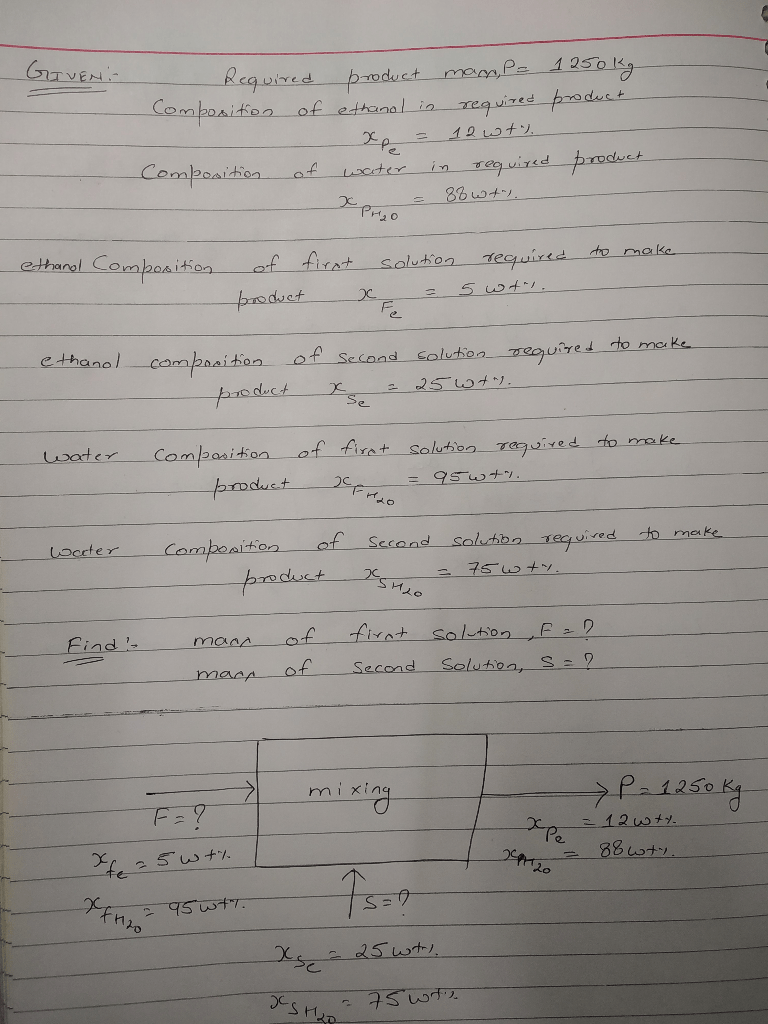# It is required to prepare 1250 kg of a solution composed of 12 wt.% ethanol and 88 wt.% water. Two solutions are available, the first contains 5 wt.% ethanol, and the second contains 25 wt.% ethanol. How much of each solution are mixed to prepare the desired solution?

Question-AnswerCategory: Material And Energy BalanceIt is required to prepare 1250 kg of a solution composed of 12 wt.% ethanol and 88 wt.% water. Two solutions are available, the first contains 5 wt.% ethanol, and the second contains 25 wt.% ethanol. How much of each solution are mixed to prepare the desired solution?

It is required to prepare 1250 kg of a solution composed of 12 wt.% ethanol and
88 wt.% water. Two solutions are available, the first contains 5 wt.% ethanol, and the second contains 25 wt.% ethanol. How much of each solution are mixed to prepare the desired solution?Solution:
1. Ethanol balance
Input = output
A (5 /100) + B( 25/ 100 ) = M ( 12 /100 )
0.05 A + 0.25 B = 0.12 M
A = (150 − 0.25 B)/ 0.05 = 3000 − 5 𝐵 … … … . . (𝟏)

2. Water balance
Input = output
0.95 A + 0.75 B = 0.88 M = 0.88 1250 = 1100
0.95 A + 0.75 B = 1100 … … … . . (𝟐)

Substituting (1) in (2)
0.95(300-5 B) + 0.75 B = 1100
2850 – 4.75 + 0.75 B =1100
4 B =1750 …………………. B= 437.5 kg
Substituting B in (1) : A= 3000 – 5(437.5) = 812.5 kg

3. Checking: Total material balance, Input = A + B = 437.5 + 812.5 =1250 kg
Output = M= 1250 kg